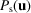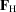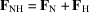International Tables for Crystallography (2010). Vol. B, ch. 2.3, pp. 244-281   | 1 | 2 | https://doi.org/10.1107/97809553602060000765

Contents

• 2.3. Patterson and molecular replacement techniques, and the use of noncrystallographic symmetry in phasing  (pp. 244-281)
• 2.3.1. Introduction  (pp. 244-247) | html | pdf |
• 2.3.1.1. Background  (pp. 244-245) | html | pdf |
• 2.3.1.2. Limits to the number of resolved vectors  (p. 245) | html | pdf |
• 2.3.1.3. Modifications: origin removal, sharpening etc .  (pp. 245-246) | html | pdf |
• 2.3.1.4. Homometric structures and the uniqueness of structure solutions; enantiomorphic solutions  (pp. 246-247) | html | pdf |
• 2.3.1.5. The Patterson synthesis of the second kind  (p. 247) | html | pdf |
• 2.3.2. Interpretation of Patterson maps  (pp. 247-251) | html | pdf |
• 2.3.2.1. Simple solutions in the triclinic cell. Selection of the origin  (pp. 247-248) | html | pdf |
• 2.3.2.2. Harker sections  (p. 248) | html | pdf |
• 2.3.2.3. Finding heavy atoms  (p. 249) | html | pdf |
• 2.3.2.4. Superposition methods. Image detection  (pp. 249-250) | html | pdf |
• 2.3.2.5. Systematic computerized Patterson vector-search procedures. Looking for rigid bodies  (p. 251) | html | pdf |
• 2.3.3. Isomorphous replacement difference Pattersons  (pp. 251-255) | html | pdf |
• 2.3.3.1. Introduction  (p. 251) | html | pdf |
• 2.3.3.2. Finding heavy atoms with centrosymmetric projections  (pp. 251-252) | html | pdf |
• 2.3.3.3. Finding heavy atoms with three-dimensional methods  (p. 252) | html | pdf |
• 2.3.3.4. Correlation functions  (pp. 252-253) | html | pdf |
• 2.3.3.5. Interpretation of isomorphous difference Pattersons  (pp. 253-254) | html | pdf |
• 2.3.3.6. Direct structure determination from difference Pattersons  (pp. 254-255) | html | pdf |
• 2.3.3.7. Isomorphism and size of the heavy-atom substitution  (p. 255) | html | pdf |
• 2.3.4. Anomalous dispersion  (pp. 255-257) | html | pdf |
• 2.3.4.1. Introduction  (pp. 255-256) | html | pdf |
• 2.3.4.2. Thefunction  (p. 256) | html | pdf |
• 2.3.4.3. The position of anomalous scatterers  (pp. 256-257) | html | pdf |
• 2.3.4.4. Computer programs for automated location of atomic positions from Patterson maps  (p. 257) | html | pdf |
• 2.3.5. Noncrystallographic symmetry  (pp. 258-260) | html | pdf |
• 2.3.5.1. Definitions  (pp. 258-259) | html | pdf |
• 2.3.5.2. Interpretation of Pattersons in the presence of noncrystallographic symmetry  (pp. 259-260) | html | pdf |
• 2.3.6. Rotation functions  (pp. 260-269) | html | pdf |
• 2.3.6.1. Introduction  (pp. 260-261) | html | pdf |
• 2.3.6.2. Matrix algebra  (pp. 262-263) | html | pdf |
• 2.3.6.3. Symmetry  (pp. 263-264) | html | pdf |
• 2.3.6.4. Sampling, background and interpretation  (pp. 264-267) | html | pdf |
• 2.3.6.5. The fast rotation function  (p. 268) | html | pdf |
• 2.3.6.6. Locked rotation functions  (pp. 268-269) | html | pdf |
• 2.3.7. Translation functions  (pp. 269-272) | html | pdf |
• 2.3.7.1. Introduction  (p. 269) | html | pdf |
• 2.3.7.2. Position of a noncrystallographic element relating two unknown structures  (p. 270) | html | pdf |
• 2.3.7.3. Position of a known molecular structure in an unknown unit cell  (pp. 270-271) | html | pdf |
• 2.3.7.4. Position of a noncrystallographic symmetry element in a poorly defined electron-density map  (p. 271) | html | pdf |
• 2.3.7.5. Locked translation function  (pp. 271-272) | html | pdf |
• 2.3.7.6. Computer programs for rotation and translation function calculations  (p. 272) | html | pdf |
• 2.3.8. Molecular replacement  (pp. 272-275) | html | pdf |
• 2.3.8.1. Using a known molecular fragment  (pp. 272-273) | html | pdf |
• 2.3.8.2. Using noncrystallographic symmetry for phase improvement  (pp. 273-274) | html | pdf |
• 2.3.8.3. Update on noncrystallographic averaging and density-modification methods  (pp. 274-275) | html | pdf |
• 2.3.8.4. Equivalence of real- and reciprocal-space molecular replacement  (p. 275) | html | pdf |
• 2.3.9. Conclusions  (p. 275) | html | pdf |
• References | html | pdf |
• Figures
• Fig. 2.3.1.1. Effect of `sharpening' Patterson coefficients  (p. 246) | html | pdf |
• Fig. 2.3.1.2. ( c ) The point Patterson of the two homometric structures in ( a ) and ( b )  (p. 246) | html | pdf |
• Fig. 2.3.2.1. Origin selection in the interpretation of a Patterson of a one-dimensional centrosymmetric structure  (p. 247) | html | pdf |
• Fig. 2.3.2.2. The c -axis projection of cuprous chloride azomethane complex (C 2 H 6 Cl 2 Cu 2 N 2 )  (p. 247) | html | pdf |
• Fig. 2.3.2.3. Atoms ABCD , arranged as a quadrilateral, generate a Patterson which is the sum of the images of the quadrilateral when each atom is placed on the origin in turn  (p. 250) | html | pdf |
• Fig. 2.3.3.1. Three different cases which can occur in the relation of the native,, and heavy-atom derivative,, structure factors for centrosymmetric reflections  (p. 251) | html | pdf |
• Fig. 2.3.3.2. Vector triangle showing the relationship between,and, where(p. 252) | html | pdf |
• Fig. 2.3.3.3. A Patterson with coefficientswill be equivalent to a Patterson whose coefficients are(p. 253) | html | pdf |
• Fig. 2.3.3.4. The phase α of the native compound (structure factor) is determined either as being equal to, or 180° out of phase with, the presumed heavy-atom contribution when only a single isomorphous compound is available  (p. 254) | html | pdf |
• Fig. 2.3.3.5. Let ( a ) be the original structure which contains three heavy atoms ABC in a noncentrosymmetric configuration  (p. 254) | html | pdf |
• Fig. 2.3.3.6. A plot of mean isomorphous differences as a function of resolution  (p. 255) | html | pdf |
• Fig. 2.3.4.1. ( a ) A model structure with an anomalous scatterer at A   (p. 256) | html | pdf |
• Fig. 2.3.4.2. Anomalous-dispersion effect for a molecule  (p. 257) | html | pdf |
• Fig. 2.3.5.1. The two-dimensional periodic design shows crystallographic twofold axes perpendicular to the page and local noncrystallographic rotation axes in the plane of the paper  (p. 258) | html | pdf |
• Fig. 2.3.5.2. The objectsandare related by an improper rotation θ   (p. 258) | html | pdf |
• Fig. 2.3.5.3. The position of the twofold rotation axis which relates the two piglets is completely arbitrary  (p. 259) | html | pdf |
• Fig. 2.3.6.1. Shape of the interference function G for a spherical envelope of radius R at a distance H from the reciprocal-space origin  (p. 261) | html | pdf |
• Fig. 2.3.6.2. Relationships of the orthogonal axesto the crystallographic axes(p. 262) | html | pdf |
• Fig. 2.3.6.3. Eulerian anglesrelating the rotated axesto the original unrotated orthogonal axes(p. 262) | html | pdf |
• Fig. 2.3.6.4. Variables ψ and ϕ are polar coordinates which specify a direction about which the axes may be rotated through an angle κ  (p. 262) | html | pdf |
• Fig. 2.3.6.5. Rotation space group diagram for the rotation function of a Pmmm Patterson functionagainst aPatterson function(p. 264) | html | pdf |
• Fig. 2.3.6.6. The locked rotation function, L , applied to the determination of the orientation of the common cold virus  (p. 267) | html | pdf |
• Fig. 2.3.7.1. Crosses represent atoms in a two-dimensional model structure  (p. 270) | html | pdf |
• Fig. 2.3.7.2. Vectors arising from the structure in Fig. 2.3.7.1  (p. 270) | html | pdf |
• Tables
• Table 2.3.1.1. Matrix representation of Patterson peaks  (p. 245) | html | pdf |
• Table 2.3.2.1. Coordinates of Patterson peaks for C 2 H 6 Cl 2 Cu 2 N 2 projection  (p. 248) | html | pdf |
• Table 2.3.2.2. Square matrix representation of vector interactions in a Patterson of a crystal with M crystallographic asymmetric units each containing N atoms  (p. 248) | html | pdf |
• Table 2.3.2.3. Position of Harker sections within a Patterson  (p. 249) | html | pdf |
• Table 2.3.5.1. Possible types of vector searches  (p. 259) | html | pdf |
• Table 2.3.5.2. Orientation of the glyceraldehyde-3-phosphate dehydrogenase molecular twofold axis in the orthorhombic cell  (p. 259) | html | pdf |
• Table 2.3.6.1. Different types of uses for the rotation function  (p. 260) | html | pdf |
• Table 2.3.6.2. Eulerian symmetry elements for all possible types of space-group rotations  (p. 264) | html | pdf |
• Table 2.3.6.3. Numbering of the rotation-function space groups  (p. 264) | html | pdf |
• Table 2.3.6.4. Rotation-function Eulerian space groups   (pp. 265-266) | html | pdf |
• Table 2.3.8.1. Molecular replacement: phase refinement as an iterative process  (p. 273) | html | pdf |